# GRU¶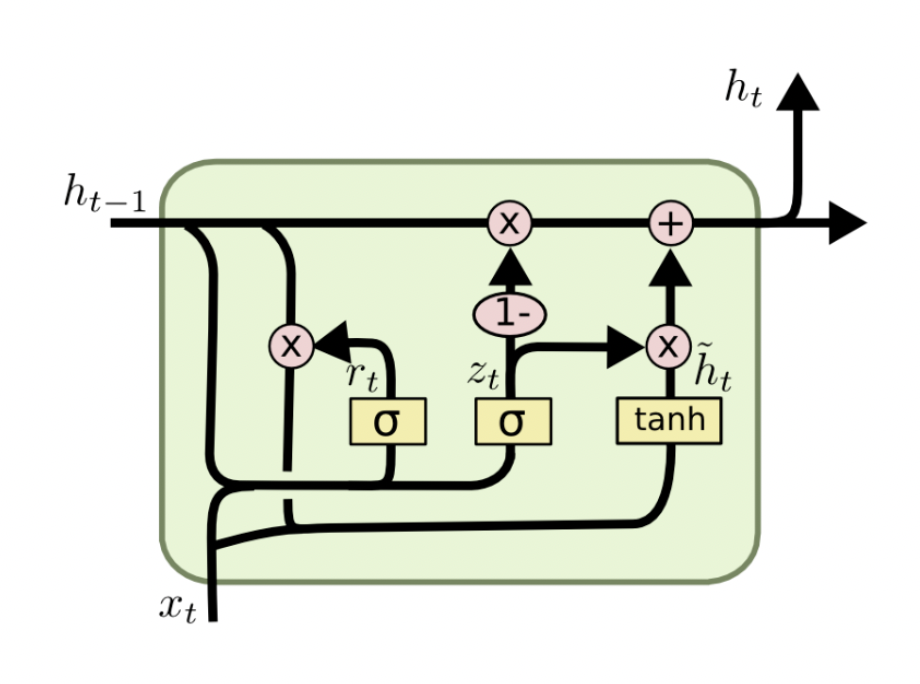GRU在LSTM的基础上主要做出了两点改变 ：

（1）GRU只有两个门。GRU将LSTM中的输入门和遗忘门合二为一，称为更新门（update gate），上图中的$$z_{t}$$，控制前边记忆信息能够继续保留到当前时刻的数据量，或者说决定有多少前一时间步的信息和当前时间步的信息要被继续传递到未来；GRU的另一个门称为重置门（reset gate），上图中的$$r_{t}$$ ，控制要遗忘多少过去的信息。

（2）取消进行线性自更新的记忆单元（memory cell），而是直接在隐藏单元中利用门控直接进行线性自更新。GRU的逻辑图如上图所示。

GRU的公式化表达如下：

$z_{t}=\sigma(W_{z} \cdot [h_{t-1},x_{t}])$
$r_{t}=\sigma(W_{r} \cdot [h_{t-1},x_{t}])$
$\tilde h=tanh(W \cdot [r_{t} \odot h_{t-1},x_{t}])$
$h_{t}=(1-z_{t})\odot h_{t-1}+z_{t} \odot \tilde h_{t-1}$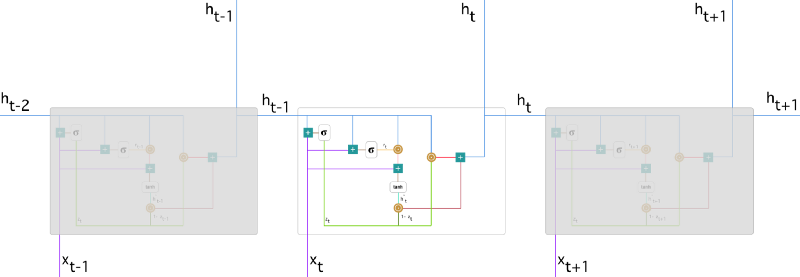## 1.更新门¶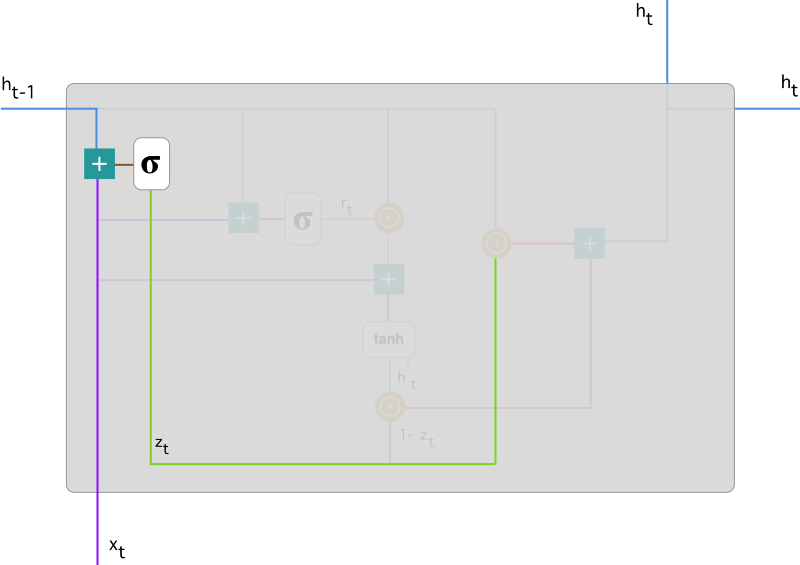## 2.重置门¶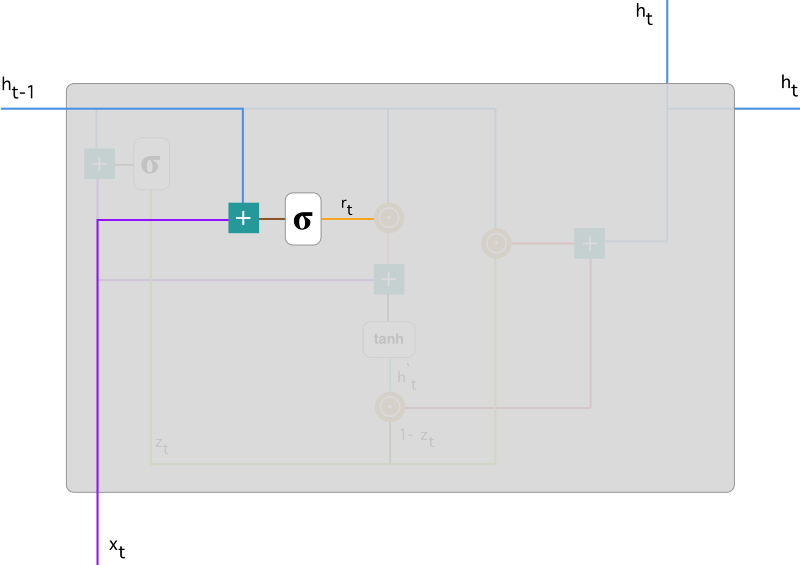## 3. 当前记忆内容¶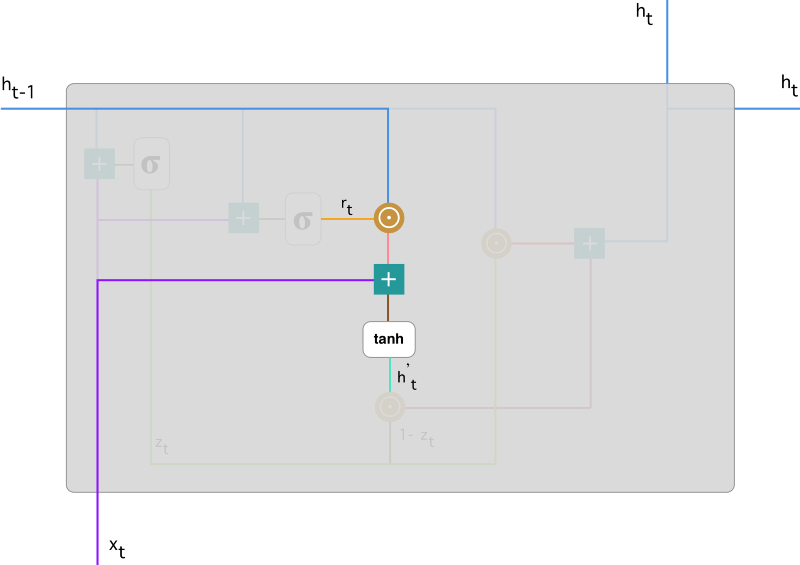## 4. 当前时间步的最终记忆¶

$h_{t}=(1-z_{t})\odot h_{t-1}+z_{t} \odot \tilde h_{t-1}$

$$z_t$$ 为更新门的激活结果，它同样以门控的形式控制了信息的流入。$$1-z_t$$$$h_{t-1}$$的 Hadamard 乘积表示前一时间步保留到最终记忆的信息，该信息加上当前记忆保留至最终记忆的信息($$z_t$$$$\tilde h_{t-1}$$的 Hadamard 乘积)就等于最终门控循环单元输出的内容。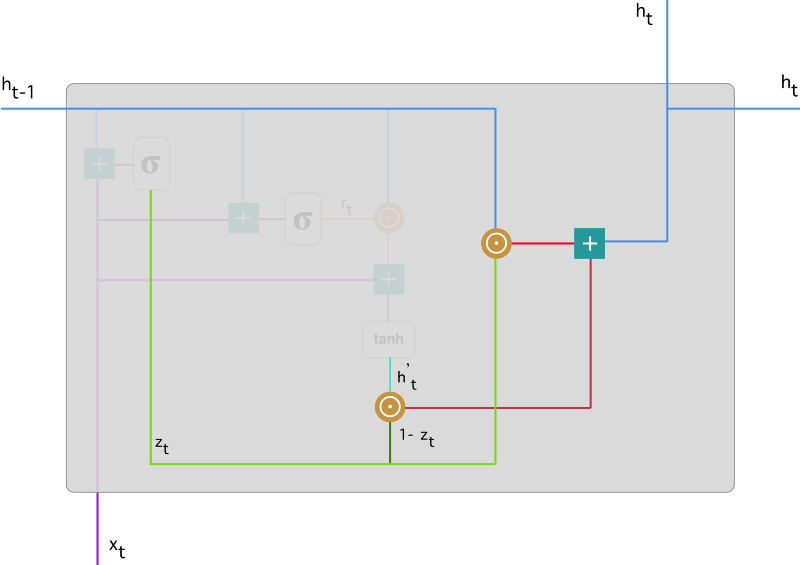## 参考文献¶

Chung, J., Gulcehre, C., Cho, K., & Bengio, Y. (2014). Empirical evaluation of gated recurrent neural networks on sequence modeling. arXiv preprint arXiv:1412.3555.链接